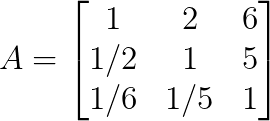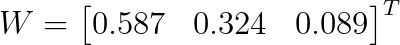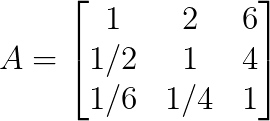# 层次分析法（AHP）分析步骤与计算方法

## # 1. 建立阶梯层次结构模型## # 2. 构造各层次中的所有判断矩阵

1. 不把所有因素放在一起比较，而是两两比较；
2. 对此时采用相对尺度，以尽可能减少性质不同的诸因素相互比较的困难，以提高准去性；

1表示两个因素相比，具有同样重要性
3表示两个因素相比，一个因素比另一个因素稍微重要
5表示两个因素相比，一个因素比另一个因素明显重要
7表示两个因素相比，一个因素比另一个因素强烈重要
9表示两个因素相比，一个因素比另一个因素极端重要
2,4,6,8上述两相邻判断的中值Z$A_1$$A_2$$A_3$
$A_1$126
$A_2$1/215
$A_3$1/61/51

## # 3. 层次单排序及其一致性检验

1. 如果成对比较矩阵是一致阵，则我们自然会取对应于最大特征根$n$ 的归一化特征向量 $\{ \omega_1,\omega_2,\omega_3,...,\omega_n\}$ ，且$\sum_{i=1}^n \omega_i = 1$$\omega_i$表示下层第 $i$个因素对上层某个因素影响程度的权值。
2. 若成对比较矩阵不是一致阵，Saaty等人建议用其最大特征根对应的归一化特征向量作为权向量 $W$ ，则$AW=\lambda W$ $W=\{ \omega_1,\omega_2,\omega_3,...,\omega_n\}$这样确定权向量的方法称为特征根法；

• $CI=0$,有完全的一致性；
• $CI$接近于0，有满意的一致性；
• $CI$越大，不一致越严重。

n1234567891011
RI000.580.901.121.241.321.411.451.491.51

### # 1).第一步：计算W1. 先对矩阵列向量归一化；

将矩阵的每一列分别加起来，再用原来的数($a_{ij}$) 除以它所在列的和。如：$1 \div (1+1/2+1/6) = 0.6$

1. 再求行和归一化；

每一行分别相加，再除以每一行的个数（即每一行的平均数）。如：$(0.6+0.615+0.545) \div 3=0.587$

​ 即得到1. 分别求出$M_1 = 1 \times 2 \times 6 = 12$$M_2 = 2$$M_3 = 0.042$

2. 计算$M_i$的n次方根：

$\overline{W_1} = \sqrt{M_1} = 2.289$$\overline{W_2} = 1.260$$\overline{W_3} = 0.347$

3. 再归一化：

$\displaystyle \sum_{i=1}^3 \overline{W_i} = 2.289+1.260+0.347 = 3.896$

$W_1 = \frac{\overline{W_1} }\sum_{i=1}^3 \overline{W_i}} = \frac{2.289}{3.896} = 0.58$$W_2 = 0.323$，$W_3 = 0.$089

### # 3).第三步：计算CI和RI

$CI=\frac {\lambda-n}{n-1} = \frac{3.009-3}{3-1} = 0.0045$

### # 4).第四步：计算CR得出结论

$CR=\frac{CI}{RI} = \frac{0.0045}{0.58}=0.0078 < 0.1$ ,通过一致性检验。

## # 4. 层次总排序及其一致性检验

• 计算某一层次所有因素对于最高层（总目标）相对重要性的权值，称为层次总排序。
• 这一过程是从最高层次到最低层次依次进行的。A层$m$个因素$A_{1},A_{2},···,A_m$,对总目标Z的排序为$a_{1},a_{2},···,a_{m}$

B层$n$个因素对上层A中因素为$A_{j}$ 的层次单排序为$b_{1j},b_{2j},···,b_{nj}(j=1,2,3,···,m)$
B层的层次总排序(即B层第$i$个因素对总目标的权值为：$\displaystyle \sum_{j=1}^{m}a_{j}b_{ij}$)为：
$B_{1}:a_{1}b_{11}+a_{2}b_{12}+···+a_{m}b_{1m}$,

$B_{2}:a_{1}b_{21}+a_{2}b_{22}+···+a_{m}b_{2m}$,
......
$B_{n}:a_{1}b_{n1}+a_{2}b_{n2}+···+a_{m}b_{nm}$,

## # 举例

某厂有一笔企业留成利润要决定如何使用，根据各方意见提出的决策方案有：发奖金；扩建集体福利设施；办技校；建图书馆；购买新设备。在决策时要考虑调动职工劳动积极性、提高职工技术文化水平、改善职工物质文化生活三方面，据此构造各因素之间相互联结的层次结构模型如下图所示。

1.建立阶梯层次结构模型2. 计算单一准则下元素的相对重要性

i. 第二层相对于第一层的判断矩阵$W=(0.105,0.637,0.258)$$λmax=3.308$

$CI=0.019$$CR=0.033<0.1$

ii. 第三层元素相对于第二层元素判断矩阵3.各个元素的权重4.结论

$W=(0.157, 0.164, 0.393, 0.113, 0.172)$

$CI=0.028$

$RI=0.923$ （这里不知道怎么来的，查表的话应该是1.12？待讨论）

$CR=0.03<0.10$

.AHP(层次分析法)方法、步骤PPT,点击下载